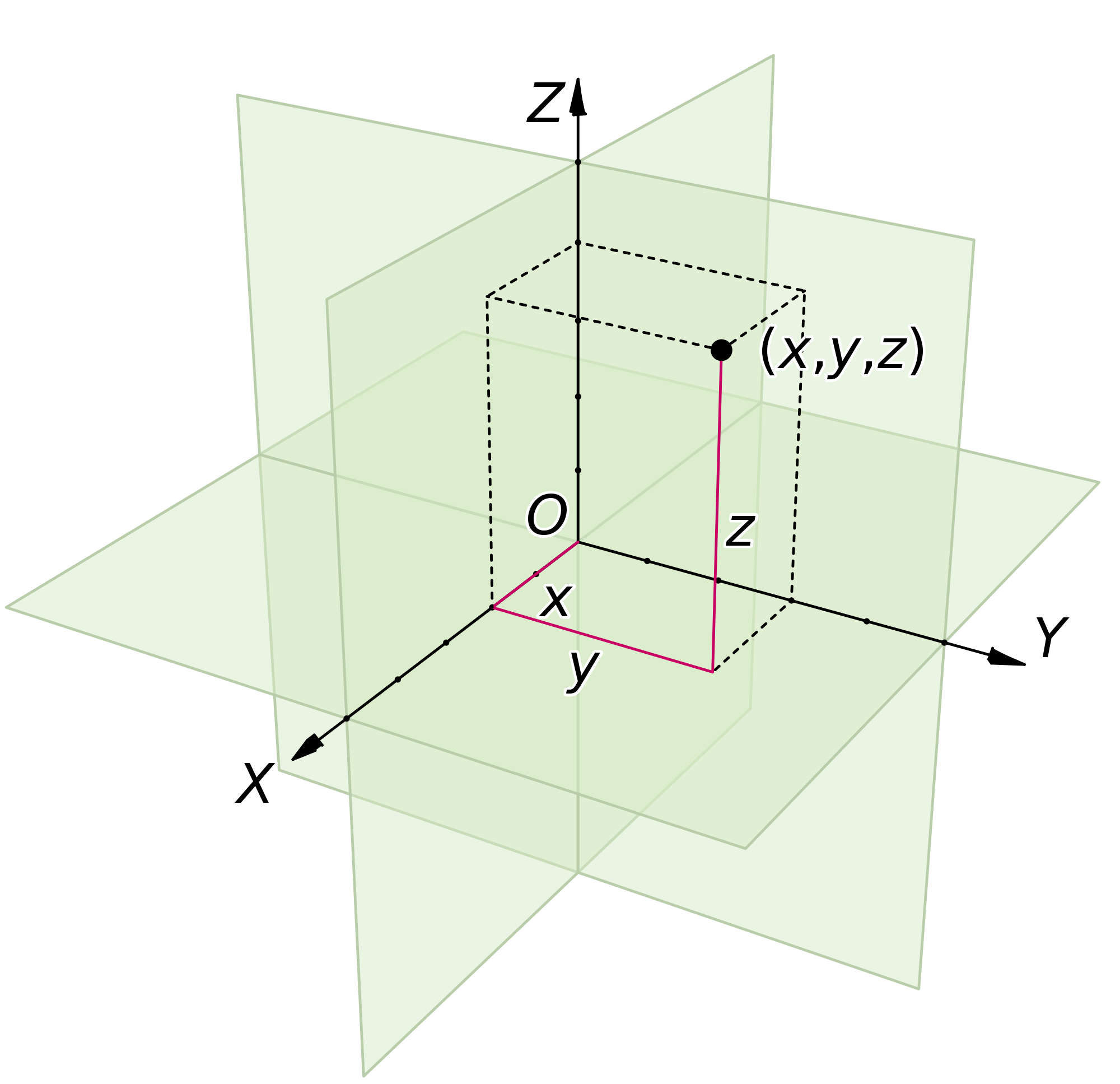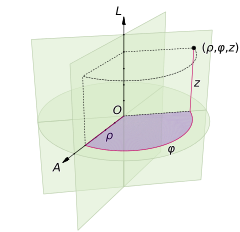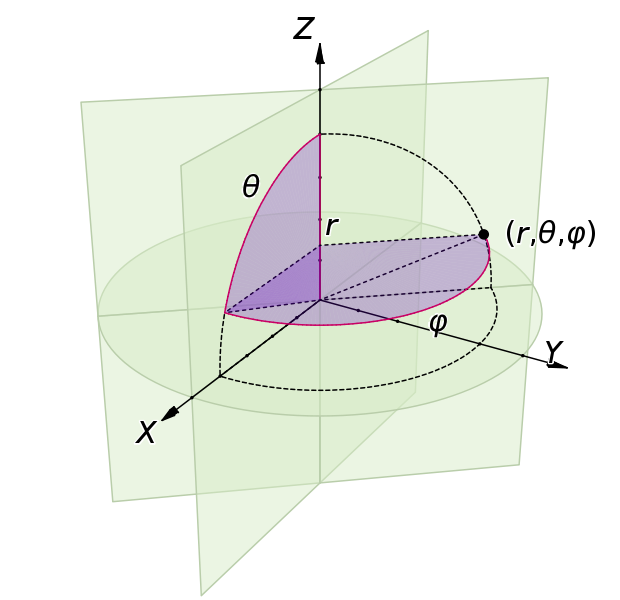### Notes of Electromagnetics [EX 503]

#### Introduction to electromagnetic

Scalar and Vector

The quantities that can be presented completely by the magnitude only are called scalars. Example : temperature, mass, density, electric potential and so on.

The quantities that require both magnitude as well as direction for their complete significance are called vectors. Example : velocity, electric field intensity, force and so on.

Vector Algebra

Assume that A and B are two vector quantities and r and s are two constants.

A + B = B + A
A + (B + C) = (A + B) + C

2. Subtraction:
A + (- B) = A - B

3. Multiplication:
(r + s) (A + B) = r A + r B + s A + s B

4. Division:
(1/r) (A + B) = A / r + B / r

Scalar Field and Vector Field

Scalar field is the field in which each point in space can be represented by scalar quantities. For example : temperature field i.e. T = e ^ (xyz / 20)

Vector field is the field in which each point in space can be represented by the vector quantities. For example : velocity field.

Dot Product

- Let us consider A and B be two vectors, then the dot product of these two vectors is represented as:
A . B = |A| * |B| * cosθ
where, θ represents the smaller angle between A and B.

- It is considered that: A . B = B . A

- Example of dot product is:
1. Work done by a force is given by:
W = F . L = F * L * cosθ = ∫ F . dL

- For A = A(x) a(x) + A(y) a(y) + A(z) a(z), where a(x), a(y) and a(z) are the unit vector components of A vector and A(x), A(y) and A(z) are constants. Similarly, for B = B(x) b(x) + B(y) b(y) + B(z) b(z)
A . B = A(x) B(x) + A(y) B(y) + A(z) B(z)

- Dot product of similar unit vectors is 1, while of dissimilar unit vectors is 0.
i.e. a(x) . a(x) = a(y) . a(y) = a(z) . a(z) = 1
a(x) . a(y) = a(y) . a(z) = a(z) . a(x) = 0

Cross Product

- For the two vectors A and B, the cross product of these two vectors is given by:
A × B = |A| * |B| * sinθ * n^

- It is perpendicular to both the vectors.

- a(x) × a(y) = a(z); a(y) × a(z) = a(x); a(z) × a(x) = a(y)
- a(y) × a(x) = -a(z); a(z) × a(y) = -a(x); a(x) × a(z) = -a(y)

- a(x) × a(x) = a(y) × a(y) = a(z) × a(z) = 0

- A × B != B × A

```     A × B =  | a(x)         a(y)          a(z)  |
|  A(x)        A(y)          A(z)  |
|  B(x)        B(y)          B(z)  |
```

Coordinate System

Rectangular Coordinate System

- It consists of three coordinate axes mutually perpendicular to each other.
- The axes are x, y and z axes.
- A point is located by its x, y and z coordinates.

- Any vector in rectangular or cartesian coordinate system can be represented by:
B = B(x) a(x) + B(y) a(y) + B(z) a(z)
where, a(x), a(y) and a(z) are the unit vectors along x, y and z axes respectively.Circular Cylindrical Component System

- It is the 3 dimensional version of polar coordinate system.
- It consists of three planes; circular cylinder (p = constant), planes (z = constant and ϕ = constant)
- The unit vectors a(p), a(z) and a(ϕ) are mutually perpendicular to each other.
- a(p) is normal to cylindrical surface, a(ϕ) is normal to plane ϕ = constant and a(z) is normal to plane z = constant.

- For the two cylinders of radius p and p + dp, two radial planes at angles ϕ and ϕ + dϕ, two horizontal planes at elevation z and z + dϕ, can enclose a small volume.
The sides are of length dp, dz and pdϕ.

So, surface areas are pdϕdp, pdϕdz and dpdz.
The volume is pdpdϕdz.Spherical Coordinate System

- It consists of three mutually perpendicular surfaces (sphere; r = constant, cone; θ = constant, and plane; ϕ = constant)
- a(r), a(θ) and a(ϕ) are unit vectors.

- Differential Elements:
The lengths are dr, rdθ and rsinθdϕRectangular and Cylindrical Coordinate Conversion

We know that:
p = √(x^2 + y^2)
ϕ = tan-1 (y / x)

Also,
x = p cosϕ
y = p sinϕ

The conversion table is shown in given table:

 a(p) a(ϕ) a(z) a(x) cosϕ - sinϕ 0 a(y) sinϕ cosϕ 0 a(z) 0 0 1

Rectangular and Spherical Coordinate Conversion

We know:
r = √(x^2 + y^2 + z^2)
θ = cos-1 (z / (√(x^2 + y^2 + z^2)))
ϕ = tan-1 (y / x)

Also,
x = r sinθ cosϕ
y = r sinθ sinϕ
z = r cosθ

The conversion table is shown in given table:

 a(r) a(θ) a(ϕ) a(x) sinθ cosϕ cosθ cosϕ - sinϕ a(y) sinθ sinϕ cosθ sinϕ cosϕ a(z) cosθ -sinθ 0

Numerical Problems of Coordinate System

1. Convert B = y a(x) - x a(y) + z a(z) into cylindrical coordinate system.

`We have, B = y a(x) - x a(y) + z a(z)`

In cylindrical system, let B = B(p) a(p) + B(ϕ) a(ϕ) + B(z) a(z)

Now, from the rectangular and cylindrical conversion table,

1. B(p) = B.a(p) = [y a(x) - x a(y) + z a(z)] . a(p)
= y a(x) . a(p) - x a(y) . a(p) + z a(z) . a(p)
= y cosϕ - x sinϕ

2. B(ϕ) = B.a(ϕ) = [y a(x) - x a(y) + z a(z)] . a(ϕ)
= y a(x) . a(ϕ) - x a(y) . a(ϕ) + z a(z) . a(ϕ)
= - y sinϕ - x cosϕ

3. B(z) = B.a(z) = z

So,
B = (y cosϕ - x sinϕ) a(p) - (y sinϕ + x cosϕ) a(ϕ) + z a(z)

Substituting value of x and y, we get:
B = (p sinϕ cosϕ - p cosϕ sinϕ) a(p) - (p (cosϕ)^2 + p (sinϕ)^2) a(ϕ) + z a(z)
= -pa(ϕ) + za(z)

2. Convert G = (xz / y) a(x) into spherical coordinate system.

```Let in spherical coordinate,
G = G(r) a(r) + G(θ) a(θ) + G(ϕ) a(ϕ)```

Now,
G(r) = G. a(r) = (xz / y) a(x) . a(r) = xz/y sinθ cosϕ
G(θ) = G. a(θ) = (xz / y) a(x) . a(θ) = xz/y cosθ cosϕ
G(ϕ) = G. a(ϕ) = (xz / y) a(x) . a(ϕ) = - xz/y sinϕ

So,
G = xz/y sinθ cosϕ a(r) + xz/y cosθ cosϕ a(θ) - xz/y sinϕ a(ϕ)

Substituting for the value of x, y and z;
G = ( r cosθ cosϕ / sinϕ) * { sinθ cosϕ a(r) + cosθ cosϕ a(θ) - sinϕ a(ϕ) }

3. Transform to cylindrical coordinates: F = 10 a(x) - 8 a(y) + 6 a(z) at point P(10, -8, 6).

```Let us consider in cylindrical coordinates:
F = F(p) a(p) + F(ϕ) a(ϕ) + F(z) a(z)```

So,
F(p) = [10 a(x) - 8 a(y) + 6 a(z)] . a(p) = 10 a(x) . a(p) - 8 a(y) . a(p) + 6 a(z) . a(p) = 10 cosϕ - 8 sinϕ
F(ϕ) = 10 a(x) . a(ϕ) - 8 a(y) . a(ϕ) + 6 a(z) . a(ϕ) = - 10 sinϕ - 8 cosϕ
F(z) = 6

Hence, F = (10 cosϕ - 8 sinϕ) a(p) - (10 sinϕ + 8 cosϕ) a(ϕ) + 6 a(z)

At point P(10, -8, 6):
p = √(x^2 + y^2) = 12.81
ϕ = tan-1 (y / x) = -38.66 degree

Now,
F = 12.81 a(p) + 6 a(z)

4. At point P(-3, -4, 5), express that vector that extends from P to Q(2, 0, -1) in spherical coordinates.

```The vector that extends from P to Q can be expressed as vector PQ given by:
PQ = 5 a(x) + 4 a(y) - 6 a(z)```

In terms of spherical coordinates:
A(r) = 5 a(x) . a(r) + 4 a(y) . a(r) - 6 a(z) . a(r) = 5 sinθ cosϕ + 4 sinθ sinϕ - 6 cosθ
A(θ) = 5 a(x) . a(θ) + 4 a(y) . a(θ) - 6 a(z) . a(θ) = 5 cosθ cosϕ + 4 cosθ sinϕ + 6 sinθ
A(ϕ) = 5 a(x) . a(ϕ) + 4 a(y) . a(ϕ) - 6 a(z) . a(ϕ) = - 5 sinϕ + 4 cosϕ

Hence,
PQ = (5 sinθ cosϕ + 4 sinθ sinϕ - 6 cosθ) a(r) + (5 cosθ cosϕ + 4 cosθ sinϕ + 6 sinθ) a(θ) + (- 5 sinϕ + 4 cosϕ) a(ϕ)

- by SURAJ AWAL